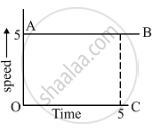Share

# A Body is Moving Uniformly in a Straight Line with a Velocity of 5 M/S. Find Graphically the Distance Covered by It in 5 Seconds. - CBSE Class 9 - Science

ConceptGraphical Representation of Motion - Velocity-time Graphs

#### Question

A body is moving uniformly in a straight line with a velocity of 5 m/s. Find graphically the distance covered by it in 5 seconds.

#### Solution

We have to calculate the distance travelled by the moving body whose speed time graph is given to us.
Distance travelled = Area of rectangle OABC
So, distance travelled,
= (OA)(OC)
= (5)(5) m
= 25 mIs there an error in this question or solution?

#### APPEARS IN

Solution A Body is Moving Uniformly in a Straight Line with a Velocity of 5 M/S. Find Graphically the Distance Covered by It in 5 Seconds. Concept: Graphical Representation of Motion - Velocity-time Graphs.
S